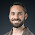Monday, January 26, 2015

Vinyls and Fourier series

Some time ago, I was talking with one of my friends about music and how vinyls are becoming popular again.

Vinyls kinda went away due to the large capacity of CDs and the increasing use of digital files, but audio enthusiasts still prefer vinyl due to fidelity.

The main difference is that vinyls use analog or continuous functions, as opposed to digital signals which have only a finite number of different states. With sound, this difference becomes more obvious depending on the sampling rate (or bit rate) of the digital signal, which basically is the length of the constant pulse. Here, having a low bit rate will make the output speaker signal to be different from the original sound, potentially loosing some part of the spectrum of the original signal.

Digital files, specially compressed files like mp3, aac, wma, etc. lose this information basically by truncating the Fourier transform of the original signal.

This loss of quality had made many people to return to the good old vinyls. These do not introduce any analog-to-digital type of loss, nor truncated spectrum.

One thing quickly came to my mind, what about stereo?

Vinyls basically give you a function $f(t)$, so how is it possible to get stereo out of this? The trick is quite simple, and it goes back to the original mechanism of how vinyls work.

The reader is a needle that moves up or down depending on the sound, that is $f(t)$
is the vertical position of the needle. Then this profile function gets wrapped into a spiral, making the disc shape of a vinyl.

But an improvement can be made to encode two signals into the devise. If you have a stereo sound made up of two functions $L(t)$ and $R(t)$, then you can set the vertical direction to be
$$y(t)=L(t)+R(t)$$
and the horizontal deflection to be
$$x(t)=L(t)-R(t)$$.

In this fashion, it is possible to encode two signals (stereo) into vinyls, having the groove to be given by the vector
$$g(t)=(x(t),y(t))$$.

Notice that this is the most we can do, as the two signals are taking 2 dimensions and time itself is represented as the third dimension (the length of the groove). So I was thinking, how about Dolby Surround? Can there be a simple solution that doesn't require to sync two turn tables?

The immediate answer is to use 3 needles instead of one. Dolby 5.1 is made up of 6 sound signals:
• Front left
• Front right
• Rear left
• Rear right
• Center
• Bass
Usually the bass is obtained out of the other 5, hence people doesn't really count it as a 6th signal. Using 3 needles and 3 encoding vectors $(x_1(t),y_1(t))\,, (x_2(t),y_2(t))\,, (x_3(t),y_3(t))$ it is possible to achieve analog Dolby surround. This would be the equivalent of having 3 turntables in sync.

But what if we wanted more channels? We could add more and more vectors, but another way of doing this would be to consider a profile function instead of individual vectors. That is, let

$$y(x,t)$$.

Here we can take $x$ to be an independent variable, where $-w\leq x\leq w$. For this, we can encode several signals $\{f_n(t)\}_{n=1}^N$ as

$$y(x,t_0)=\sum_{n=1}^N \frac{1}{\sqrt{w}}f_n(t_0)\cos\left(\frac{n\pi}{w}x\right)$$.

The idea is to use Fourier series as a way to expand the idea of using a vector space to put the information in vector form. By doing this, we avoid the need of using more and more spacial vectors to represent the signals, but put the information in a profile function.

Then, for each $t_0$, $y(x,t_0)$ encodes $N$ numbers which can be recovered by performing the infer product with the desired channel

$$\langle y(x,t_0), \phi_n(x)\rangle=\int_{-w}^w y(x,t_0) \phi_n(x)\, dx$$

with

$$\phi_n(x)=\frac{1}{\sqrt{w}}\cos\left(\frac{n\pi}{w}x\right)$$.

With this procedure, it is possible to encode any countable number of signals into a vinyl, just probably restricted up to the boundedness of the functions.

In practice, this solution can be a little troublesome since it needs a continuous transducer in the transverse direction, which can be very difficult to achieve without relying on some kind of quantization.

1.1.2.$f_n$ son las señales que queremos combinar. Al principio debemos de fijar el número de canales $n$. Las $f_n$ son las distintas señales provenientes de los $n$ canales que fijamos y al combinarlas obtenemos el perfil del vinil, $y(x,t)$.